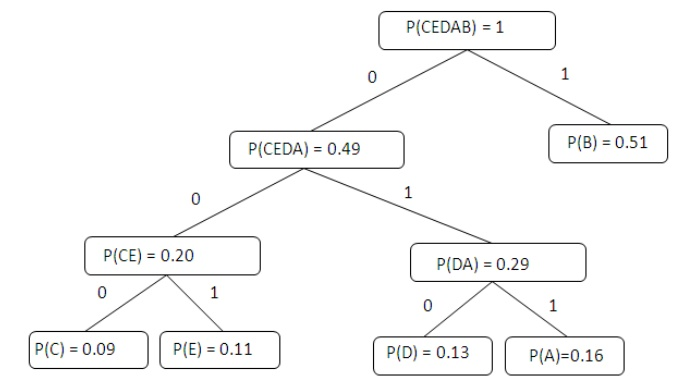Home | | Digital Communication | Source Coding

# Source Coding

A code is defined as an n-tuple of q elements. Where q is any alphabet. Ex. 1001 n=4, q={1,0} Ex.

SOURCE CODING:

A code is defined as an n-tuple of q elements. Where q is any alphabet. Ex. 1001 n=4, q={1,0} Ex.

2389047298738904 n=16, q={0,1,2,3,4,5,6,7,8,9} Ex. (a,b,c,d,e) n=5, q={a,b,c,d,e,…,y,z} The most common code is when q={1,0}. This is known as a binary code. The purpose A message can become distorted through a wide range of unpredictable errors.

Humans

Equipment failure

Lighting interference

Scratches in a magnetic tape

Ø   Error-correcting code:

To add redundancy to a message so the original message can be recovered if it has been

garbled. e.g. message = 10 code = 1010101010

Ø   Send a message:Ø   Source Coding loss:

It may consider semantics of the data depends on characteristics of the data e.g. DCT, DPCM, ADPCM, color model transform A code is distinct if each code word can be distinguished from every other (mapping is one-to-one) uniquely decodable if every code word is identifiable when immersed in a sequence of code words e.g., with previous table, message 11 could be defined as either ddddd or bbbbbb Measure of Information Consider symbols si and the probability of occurrence of each symbol p(si)

Example Alphabet = {A, B} p(A) = 0.4; p(B) = 0.6 Compute Entropy (H) -0.4*log2 0.4 + - 0.6*log2 0.6 = .97 bits Maximum uncertainty (gives largest H) occurs when all probabilities are equal Redundancy Difference between avg. codeword length (L) and avg. information content (H) If H is constant, then can just use L Relative to the optimal value

Ø   Shannon-Fano Algorithm:

Arrange the character set in order of decreasing probability

While a probability class contains more than one symbol:

ü   Divide the probability class in two so that the probabilities in the two halves are as nearly as possible equal.

ü   Assign a '1' to the first probability class, and a '0' to the second

TABLE : 5.1Ø   Huffman Encoding:

Statistical encoding To determine Huffman code, it is useful to construct a binary tree Leaves are characters to be encoded Nodes carry occurrence probabilities of the characters belonging to the subtree Example: How does a Huffman code look like for symbols with statistical symbol occurrence probabilities: P(A) = 8/20, P(B) = 3/20, P(C ) = 7/20, P(D) = 2/20? Step 1 : Sort all Symbols according to their probabilities (left to right) from Smallest to largest these are the leaves of the Huffman tree Step 2: Build a binary tree from left toRight Policy: always connect two smaller nodes together (e.g., P(CE) and P(DA) had both Probabilities that were smaller than P(B), Hence those two did connect first Step 3: label left branches of the tree With 0 and right branches of the tree With 1 Step 4: Create Huffman Code Symbol A = 011 Symbol B = 1 Symbol C = 000 Symbol D = 010 Symbol E = 001Study Material, Lecturing Notes, Assignment, Reference, Wiki description explanation, brief detail
Communication Theory : Information Theory : Source Coding |